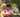### JavaScript Math Object

January 15, 2020

JavaScript Math Object

Math is a built-in object that has properties and methods for mathematical constants and functions. Not a function object. Math works with the Number type. It doesn't work with BigInt which is a built-in object that provides a way to represent whole numbers larger than 2**53 - 1.

Unlike the other global objects, Math is not a constructor. All properties and methods of Math are static. You refer to the constant pi as Math.PI and you call the sine function as Math.sin(x), where x is the method's argument. Constants are defined with the full precision of real numbers in JavaScript.

### Properties

Math.E : Euler's constant and the base of natural logarithms; approximately 2.718.
Math.LN2 : Natural logarithm of 2; approximately 0.693.
Math.LN10 : Natural logarithm of 10; approximately 2.303.
Math.LOG2E : Base 2 logarithm of E; approximately 1.443.
Math.LOG10E : Base 10 logarithm of E; approximately 0.434.
Math.PI : Ratio of the a circle's circumference to its diameter; approximately 3.14159.
Math.SQRT1_2 : Square root of ½ (or equivalently, 1/√2 over the square root of 2); approximately 0.707.
Math.SQRT2 : Square root of 2; approximately 1.414.

### Methods

Math.abs(x)
Returns the absolute value of a number.

``````function difference(a, b) {
return Math.abs(a - b);
}
console.log(difference(3, 5)); // expected output: 2
console.log(difference(5, 3)); // expected output: 2
console.log(difference(1.23456, 7.89012)); // expected output: 6.6555599999999995``````

Math.ceil(x)
Returns the smallest integer greater than or equal to a number.

``````console.log(Math.ceil(.95)); // expected output: 1
console.log(Math.ceil(4)); // expected output: 4
console.log(Math.ceil(7.004)); // expected output: 8
console.log(Math.ceil(-7.004)); // expected output: -7``````

Math.floor(x)
Returns the largest integer less than or equal to a number.

``````console.log(Math.floor(5.95)); // expected output: 5
console.log(Math.floor(5.05)); // expected output: 5
console.log(Math.floor(5)); // expected output: 5
console.log(Math.floor(-5.05));// expected output: -6``````

Math.round(x)
Returns the value of a number rounded to the nearest integer.

``````console.log(Math.round(0.9)); // expected output: 1
console.log(Math.round(5.95), Math.round(5.5), Math.round(5.05));
// expected output: 6 6 5
console.log(Math.round(-5.05), Math.round(-5.5), Math.round(-5.95));
// expected output: -5 -5 -6``````

Math.random()
Returns a pseudo-random number between 0 and 1.

``````function getRandomInt(max) {
return Math.floor( Math.random() * Math.floor(max) );
}
console.log(getRandomInt(3)); // expected output: 0, 1 or 2
console.log(getRandomInt(1)); // expected output: 0
console.log(Math.random()); // expected output: a number between 0 and 1``````

Math.trunc(x)
Returns the integer part of the number x, removing any fractional digits.

``````console.log(Math.trunc(13.37)); // expected output: 13
console.log(Math.trunc(42.84)); // expected output: 42
console.log(Math.trunc(0.123)); // expected output: 0
console.log(Math.trunc(-0.123)); // expected output: -0``````

Math.max([x[, y[, …]]])
Returns the largest of zero or more numbers.

``````console.log( Math.max(1, 3, 2) ); // expected output: 3
console.log( Math.max(-1, -3, -2) ); // expected output: -1
const array1 = [1, 3, 2];
console.log( Math.max(...array1) ); // expected output: 3``````

Math.min([x[, y[, …]]])
Returns the smallest of zero or more numbers.

``````console.log(Math.min(2, 3, 1)); // expected output: 1
console.log(Math.min(-2, -3, -1)); // expected output: -3
const array1 = [2, 3, 1];
console.log(Math.min(...array1)); // expected output: 1``````Posted by Tai Lu ( 呂台生 ) who's building useful things.You should follow him on Twitter